# Introduction

Load the library, a few density profiles and try to trim them automatically:

library(densitr)
dp.list <- dpload(dp.directory = system.file("extdata", package = "densitr"))
dp.trimmed  <- dptriml(dp.list)
#> started trimming 15 files
#> ########################################
#> trimming report:
#> analysed 15 file(s)
#> start detection failed in: 0 file(s)
#> end detection failed in: 6 file(s).
#> ########################################
#> end fail(s):
#> 00050045, 00050046, 00050012, 00050013, 00050036, 00050038

You can either trim failed density profiles by hand or just check the failures by plotting them and deciding to ignore them, as demonstrated in vignette “Manual trimming of density profiles”.

# Detrending density profiles

When drilling into wood and recording resistance to drilling, there is an underlying trend to values from those measurements. This is most likely due to the increased friction as the drilling needle is drilling deeper, but other explanations are also possible. Whatever the case, some people (including the author of this package) consider detrending the measurements essential before calculating any derived values from density profiles.

Detrending work by fitting a function to density profiles and substracting those values from the original values. The workhorse for this in densitr is function dpdetrend, which takes a density profile, detrends it using the specified function and returns the modified density profile.

Two detrending functions are currently implemented: linear regression and generalized additive model (GAM). The latter requires the package mcgv to work, the default smoothing parameter estimation method is “REML”, as it is a relatively safe choice - see mgcv::gam documentation for more. It is also easy to implement your own detrending functions using lapply on a list of density profiles.

Detrending should be done on trimmed profiles, otherwise the starting/ending portion of the profile will influence the fit and therefore the results. This is the original density profile:

plot(dp.list[])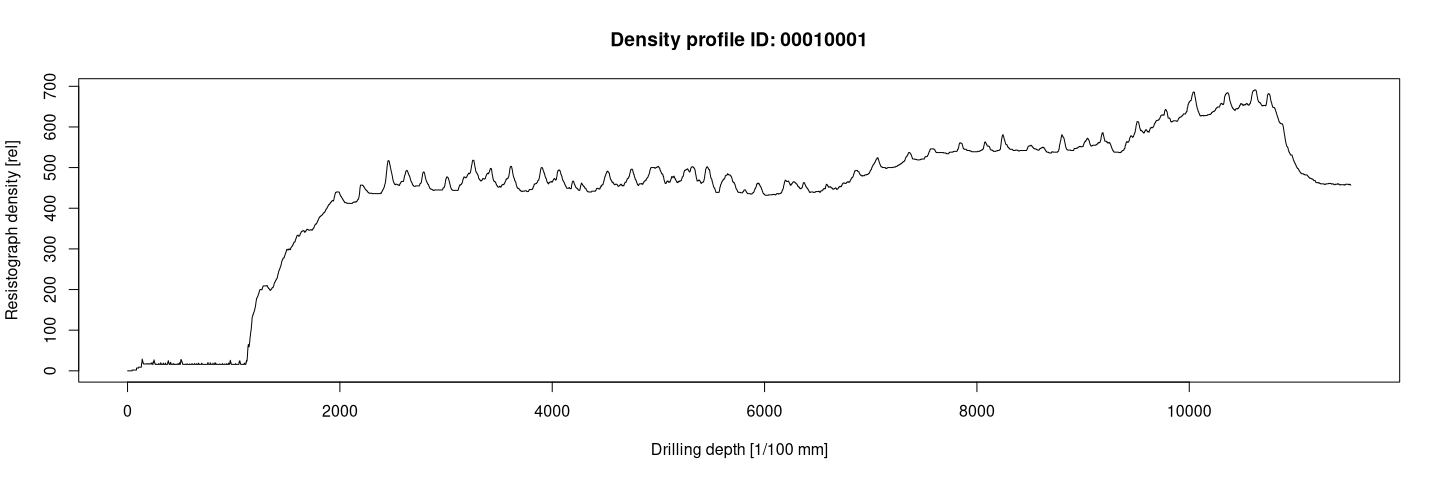And this is the trimmed one:

plot(dp.trimmed[])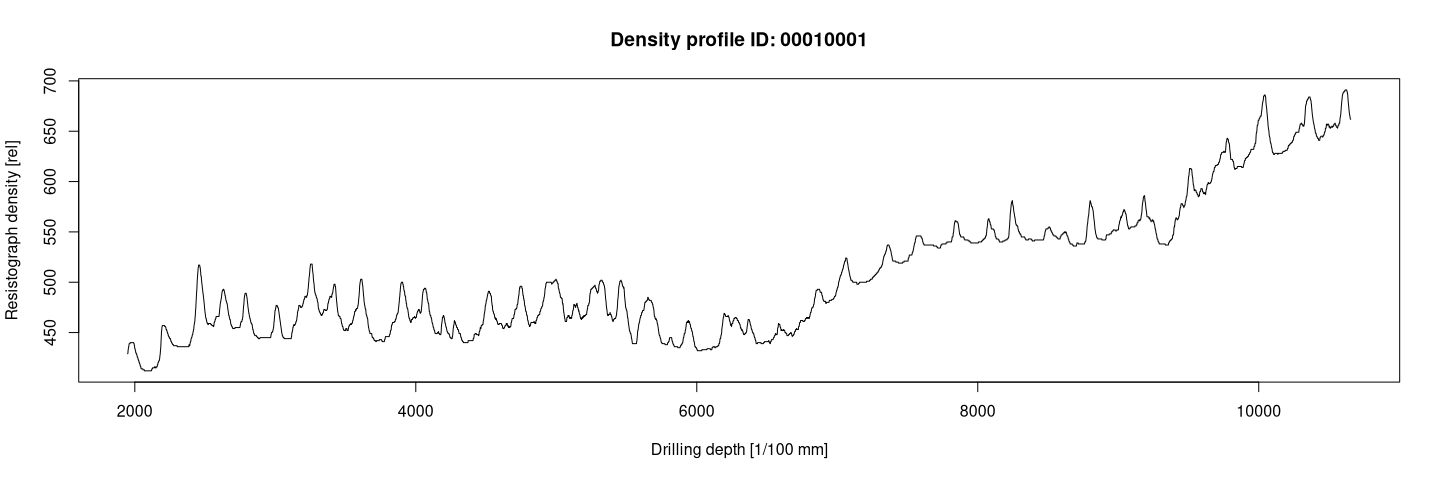## Detrending using linear regression

Let’s detrend one profile manually in order to demonstrate the process:

trend <- stats::lm(amplitude ~ position, data = dp.trimmed[]$data) fit <- stats::predict(trend, newdata = dp.trimmed[]$data)
detrended.amplitude  <- dp.trimmed[]$data$amplitude - fit + fit

Plot the results:

plot(y = dp.trimmed[]$data$amplitude,
x = dp.trimmed[]$data$position,
type = "l", lty = 1, ylim = c(350,700),
xlab = "Drilling depth [1/100 mm]",
ylab = "Resistograph density [rel]")
abline(trend, lty=2, col = "blue")
lines(y = detrended.amplitude, x = dp.trimmed[]$data$position, ylim = c(100, 700), col = "red")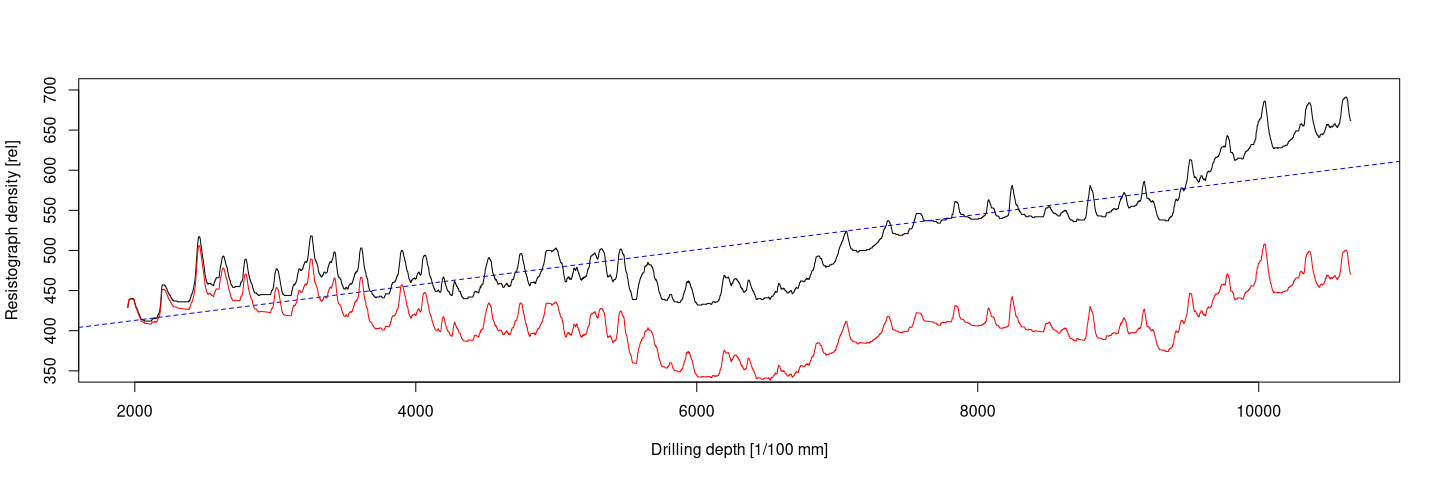The same result can be obtained by calling dpdetrend(..., type = "linear") on individual profiles.

## Detrending using GAM

This method can be used to remove all trends from the profile, just call dpdetrend with the argument type = "gam". Example of a detrended profile from above:

dp.detrended  <- dpdetrend(dp.trimmed[], type = "gam")
plot(dp.detrended)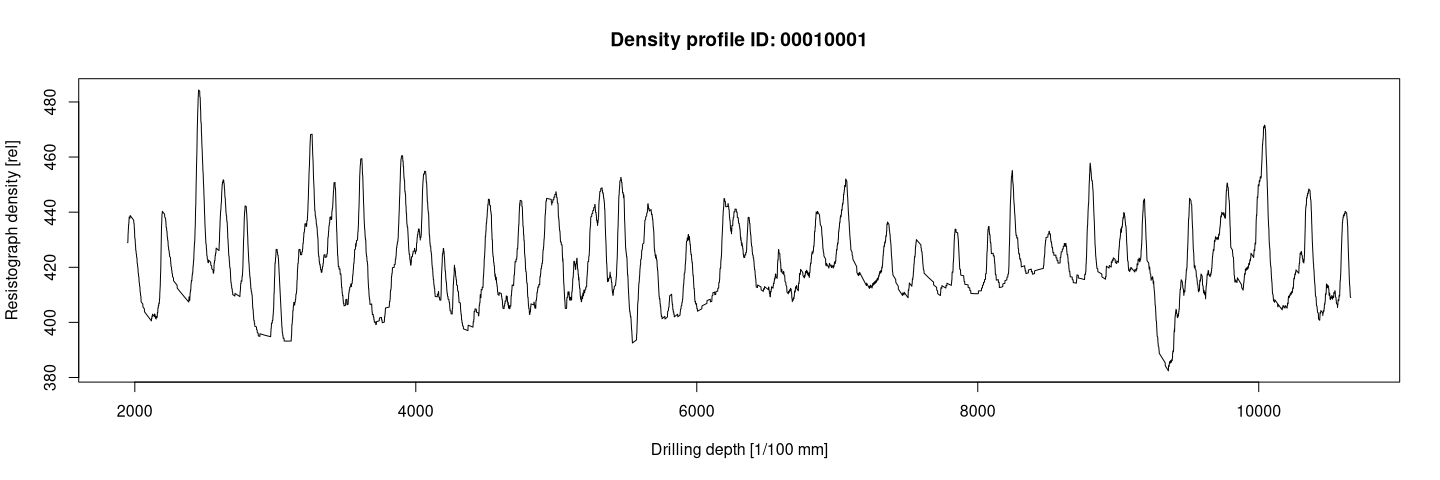Comparison of non-detrended (black line), linear-detrended (red line) and gam-detrended (blue line) profile :

plot(y = dp.trimmed[]$data$amplitude,
x = dp.trimmed[]$data$position,
type = "l", lty = 1, ylim = c(350,700),
xlab = "Drilling depth [1/100 mm]",
ylab = "Resistograph density [rel]")
lines(y = detrended.amplitude, x = dp.trimmed[]$data$position, ylim = c(100, 700), col = "red")
lines(y = dp.detrended$data$amplitude, x = dp.detrended$data$position, ylim = c(100, 700), col = "blue")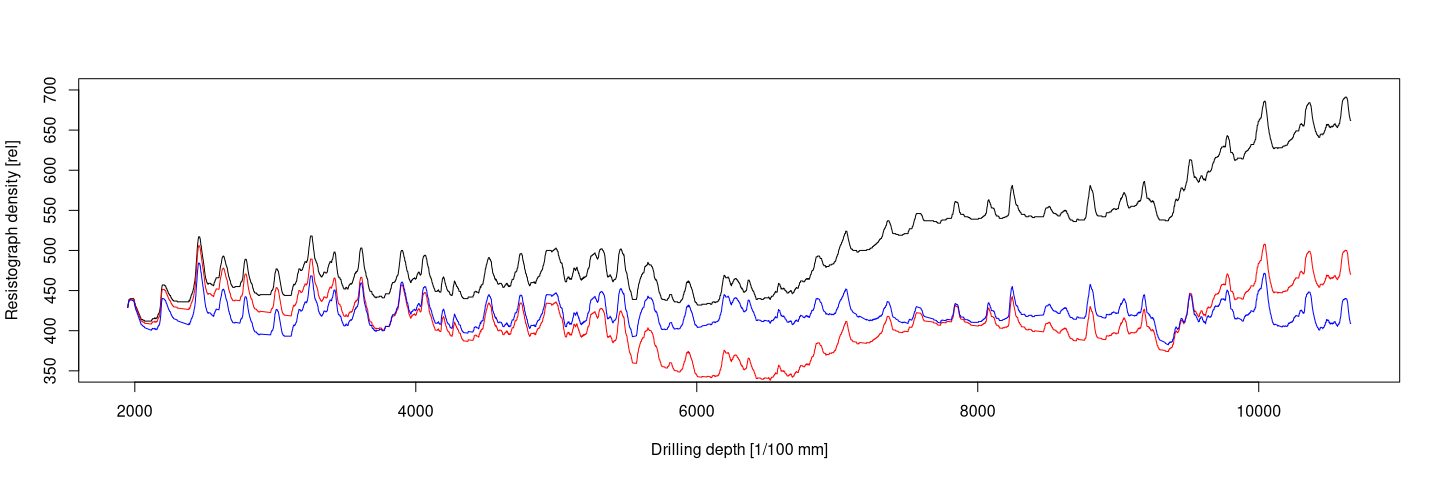## Detrending a list To detrend a list of density profiles use either lapply or pblapply, which take a function and apply it to each item in a list. pblapply can run on multiple cores (by specifying cl = 4, it will run on 4 cores in parallel. This can speed up the detrending process significantly. Other functions such as dtriml and dtriml_s will use pbapply if present - you can also run them in parallel.

For example, to detrend a list running on 3 cores:

library(pbapply)
dp.detrended  <- pblapply(dp.trimmed, dpdetrend, type = "linear", cl=3)
## without pbapply library, you could just use:
dp.detrended  <- lapply(dp.trimmed, dpdetrend, type = "linear")